# Naming polygons Online Quiz

#### Complete Python Prime Pack

9 Courses     2 eBooks

#### Artificial Intelligence & Machine Learning Prime Pack

6 Courses     1 eBooks

#### Java Prime Pack

9 Courses     2 eBooks

Following quiz provides Multiple Choice Questions (MCQs) related to Naming polygons. You will have to read all the given answers and click over the correct answer. If you are not sure about the answer then you can check the answer using Show Answer button. You can use Next Quiz button to check new set of questions in the quiz.Q 1 - Name the following polygon based on its number of sides.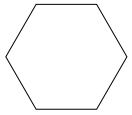### Explanation

Step 1:

Given polygon has six sides.

Step 2:

So it is a Hexagon.

Q 2 - Name the following polygon based on its number of sides.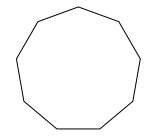### Explanation

Step 1:

Given polygon has nine sides.

Step 2:

So it is a Nonagon.

Q 3 - Name the following polygon based on its number of sides.### Explanation

Step 1:

Given polygon has four sides.

Step 2:

So it is a tetragon or quadrilateral. It is special tetragon named Rectangle.

Q 4 - Name the following polygon based on its number of sides.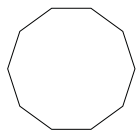### Explanation

Step 1:

Given polygon has ten sides.

Step 2:

So it is a Decagon.

Q 5 - Name the following polygon based on its number of sides.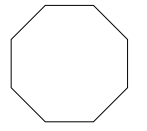### Explanation

Step 1:

Given polygon has eight sides.

Step 2:

So it is a Octagon.

Q 6 - Name the following polygon based on its number of sides.### Explanation

Step 1:

Given polygon has three sides.

Step 2:

So it is a trigon or a Triangle.

Q 7 - Name the following polygon based on its number of sides.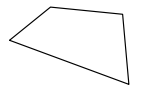### Explanation

Step 1:

Given polygon has four sides.

Step 2:

So it is a tetragon or quadrilateral.

Q 8 - Name the following polygon based on its number of sides.### Explanation

Step 1:

Given polygon has seven sides.

Step 2:

So it is a Heptagon.

Q 9 - Name the following polygon based on its number of sides.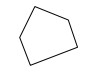### Explanation

Step 1:

Given polygon has five sides.

Step 2:

So it is a Pentagon.

Q 10 - Name the following polygon based on its number of sides.### Explanation

Step 1:

Given polygon has four sides.

Step 2:

So it is a tetragon or quadrilateral. It is special tetragon named Trapezium.

naming_polygons.htm